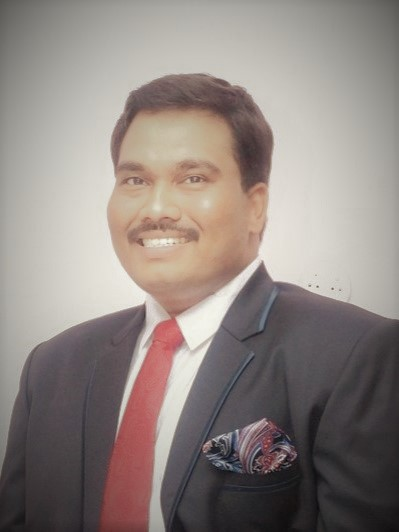# Network AnalysisTeacher

##### Nanda Kishore RayCategory

### Course Attendees

Still no participant

Still no reviews

# Code(Credit) : CUTM1043 (2-1-0)

## Course Objectives

• To learn techniques of solving circuits involving different active and passive elements.
• To analyze the behavior of the circuit’s response in time domain.
• To analyze the behavior of the circuit’s response in frequency domain.
• To synthesize an electrical network from a given impedance/admittance function.

## Learning Outcomes

• Apply the knowledge of basic circuital law and simplify the network using reduction techniques.
• Analyze the circuit using Kirchhoff’s law and Network simplification theorems.
• Infer and evaluate transient response, Steady state response, network functions.
• Obtain the maximum power transfer to the load , and Analyze the series resonant and parallel resonant circuit.
• Evaluate two-port network parameters.

## Course Syllabus

Module I: Basic Circuit Concepts and Basic Laws (3hrs Theory)
Circuit concepts –Resistor(R)-Inductor(L)-Capacitor(C)-Voltage and Current Sources - Source transformation-Voltage, Current relationship for passive bilateral elements -Ohm’s law, Kirchhoff’s laws.

Module II: Methods of Analysis (3hrs Theory)
Nodal analysis, mesh analysis, super node and super mesh, Nodal Versus Mesh Analysis for D.C excitations.

Module III: Circuit Theorems (3hrs Theory + 4hr Practice)
Superposition theorem- Thevenin’s theorem- Norton’s theorem- Maximum power transfer theorem- Reciprocity theorem.

Practice:
1. Verification of Superposition Theorem
2. Verification of Thevenin's Theorem
3. Verification of Maximum Power Transfer Theorem
4. Verification of Reciprocity Theorem

Module IV: NETWORK TOPOLOGY AND TWO PORT NETWORKS (3hrs Theory + 4hrs Practice)
Network topology, Incidence matrix, Tie-set matrix, Cut-set matrix, Dual networks- Two port network, Impedance Parameter, Admittance Parameter, Transmission line.

Practice:
1. Determination of Z parameters of Port Network
2. Determination of Y parameters of Port Network
3. Determination of h parametersof Port Network
4. Determination of ABCD parametersof Port Network

Module V: Time Response of Circuits (DC Excitation) (3hrs Theory)
Time (Transient) response of R-L, R-C, R-L-C series circuits for Zero input, Step input, pulse input -Initial conditions-solution method using differential equation and Laplace transforms.

Module VI: Single Phase A.C Circuits:(3hrs Theory + 3hrs Practice)
Sinusoidal alternating quantities – Phase and Phase difference – Complex and polar forms of representations, J-notation, R.M.S, Average values and form factor for different periodic wave forms - Steady state analysis of R,L and C (in series, parallel and series parallel combinations) with sinusoidal excitation-Concept of Reactance, Impedance Susceptance and Admittance-Power Factor and significance-Real and Reactive power, Complex Power.

Practice:
1. Study of Step Response of R-L Network
2. Study of Step Response of R-L Network
3. Study of Time Response of R-L-C Network

Module VII:
S Domain Analysis (3hrs Theory + 1hr Practice)

Transform Impedance and Transform Circuits, Series and Parallel Combination of Elements, Terminal Pairs, Network Function for the One port and Two port, Poles and Zeros of Network Functions.

Practice:
1. Frequency response of a series and parallel resonant circuit by laboratory set up

Text Books:
1. 1. M. E. VAN VALKENBURG, “Network Analysis”, PHI Publications.
2. A Sudhakar and Shyammhoan S Palli, “Network Analysis”, MC Graw Hill Publishers.

Reference:
1. Smarajit Ghosh, “Network Theory Analysis & Synthesis”, MC Graw Hill Publishers.
2. B.R.GUPTA, "Network Analysis & Synthesis", S.Chand.
3. BH ferri, "Linear Circuits 2: AC Analysis", Coursera
4. B Tapas Kumar, "Linear Analysis", NPTEL

### Nanda Kishore Ray

##### Teacher
VIEW PROFILE### Dr P Anthony Sunny Dayal

##### Associate Professor, Department of Electronics and Communication Engineering, SOET
VIEW PROFILE

Dr P Anthony Sunny Dayal completed his M.Tech in Radar and Microwave Engineering from Andhra University and Ph.D in Antenna Array Design For Radar And Emc Applications from Centurion University. Recipient of “Young Scientist Award” from His Excellency Shri Biswabhusan Harichandan Governor of Andhra Pradesh, at Andhra Pradesh Science Congress – 2019 by Andhra Pradesh […]